对抗神经网络_keras 对抗神经网络 - CSDN
• 对抗神经网络其实是两个网络的组合，可以理解为一个网络生成模拟数据，另一个网络判断生成的数据是真实的还是模拟的。生成模拟数据的网络要不断优化自己让判别的网络判断不出来，判别的网络也要不断优化自己让判断的...
      对抗神经网络其实是两个网络的组合，可以理解为一个网络生成模拟数据，另一个网络判断生成的数据是真实的还是模拟的。生成模拟数据的网络要不断优化自己让判别的网络判断不出来，判别的网络也要不断优化自己让判断的更加精确。两者的关系形成对抗，因此叫对抗神经网络。

GAN由generator（生成模型）和discriminator（判别式模型）两部分构成。

generator：主要是从训练数据中产生相同分布的samples，对于输入x，类别标签y，在生成模型中估计其联合概率分布。

discriminator：判断输入的是真实数据还是generator生成的数据，即估计样本属于某类的条件概率分布。它采用传统的监督学习的方法。

二者结合之后，经过大量次数的迭代训练会使generator尽可能模拟出以假乱真的样本，而discrimator会有更精确的鉴别真伪数据的能力，最终整个GAN会达到所谓的纳什均衡，即discriminator对于generator的数据鉴别结果为正确率和错误率各占50%。

GAN的实现，本例以mnist数据为例，直接代码

进行训练

# 定义load_data()函数以读取数据
'''
函数功能：导出MNIST数据
输入: data_path   传入数据所在路径（解压后的数据）
输出: train_data  输出data，形状为(60000, 28, 28, 1)
train_label  输出label，形状为(60000, 1)
'''

f_data = open(os.path.join(data_path, 'train-images.idx3-ubyte'))
# 前16个字符为说明符，需要跳过

f_label = open(os.path.join(data_path, 'train-labels.idx1-ubyte'))
# 前8个字符为说明符，需要跳过

return train_data, train_label

# 导入需要的包
import os  # 读取路径下文件
import shutil  # 递归删除文件
import tensorflow as tf  # 编写神经网络
import numpy as np  # 矩阵运算操作
from skimage.io import imsave  # 保存影像
from tensorflow.examples.tutorials.mnist import input_data  # 第一次下载数据时用

# 图像的size为(28, 28, 1)
image_height = 28
image_width = 28
image_size = image_height * image_width

# 是否训练和存储设置
train = True
restore = False  # 是否存储训练结果
output_path = "./output/"  # 存储文件的路径

# 实验所需的超参数
max_epoch = 500
batch_size = 256
h1_size = 256  # 第一隐藏层的size，即特征数
h2_size = 512  # 第二隐藏层的size，即特征数
z_size = 128  # 生成器的传入参数

# 导入tensorflow
import tensorflow as tf

# 定义GAN的生成器
def generator(z_prior):
'''
函数功能：生成影像，参与训练过程
输入：z_prior,       #输入tf格式，size为（batch_size, z_size）的数据
输出：x_generate,    #生成图像
g_params,      #生成图像的所有参数
'''
# 第一个链接层
# 以2倍标准差stddev的截断的正态分布中生成大小为[z_size, h1_size]的随机值，权值weight初始化。
w1 = tf.Variable(tf.truncated_normal([z_size, h1_size], stddev=0.1), name="g_w1", dtype=tf.float32)
# 生成大小为[h1_size]的0值矩阵，偏置bias初始化
b1 = tf.Variable(tf.zeros([h1_size]), name="g_b1", dtype=tf.float32)
# 通过矩阵运算，将输入z_prior传入隐含层h1。激活函数为relu
h1 = tf.nn.relu(tf.matmul(z_prior, w1) + b1)

# 第二个链接层
# 以2倍标准差stddev的截断的正态分布中生成大小为[h1_size, h2_size]的随机值，权值weight初始化。
w2 = tf.Variable(tf.truncated_normal([h1_size, h2_size], stddev=0.1), name="g_w2", dtype=tf.float32)
# 生成大小为[h2_size]的0值矩阵，偏置bias初始化
b2 = tf.Variable(tf.zeros([h2_size]), name="g_b2", dtype=tf.float32)
# 通过矩阵运算，将h1传入隐含层h2。激活函数为relu
h2 = tf.nn.relu(tf.matmul(h1, w2) + b2)

# 第三个链接层
# 以2倍标准差stddev的截断的正态分布中生成大小为[h2_size, image_size]的随机值，权值weight初始化。
w3 = tf.Variable(tf.truncated_normal([h2_size, image_size], stddev=0.1), name="g_w3", dtype=tf.float32)
# 生成大小为[image_size]的0值矩阵，偏置bias初始化
b3 = tf.Variable(tf.zeros([image_size]), name="g_b3", dtype=tf.float32)
# 通过矩阵运算，将h2传入隐含层h3。
h3 = tf.matmul(h2, w3) + b3
# 利用tanh激活函数，将h3传入输出层
x_generate = tf.nn.tanh(h3)

# 将所有参数合并到一起
g_params = [w1, b1, w2, b2, w3, b3]

return x_generate, g_params

# 定义GAN的判别器
def discriminator(x_data, x_generated, keep_prob):
'''
函数功能：对输入数据进行判断，并保存其参数
输入：x_data,        #输入的真实数据
x_generated,     #生成器生成的虚假数据
keep_prob，      #dropout率，防止过拟合
输出：y_data,        #判别器对batch个数据的处理结果
y_generated,     #判别器对余下数据的处理结果
d_params，       #判别器的参数
'''

# 合并输入数据，包括真实数据x_data和生成器生成的假数据x_generated
x_in = tf.concat([x_data, x_generated], 0)

# 第一个链接层
# 以2倍标准差stddev的截断的正态分布中生成大小为[image_size, h2_size]的随机值，权值weight初始化。
w1 = tf.Variable(tf.truncated_normal([image_size, h2_size], stddev=0.1), name="d_w1", dtype=tf.float32)
# 生成大小为[h2_size]的0值矩阵，偏置bias初始化
b1 = tf.Variable(tf.zeros([h2_size]), name="d_b1", dtype=tf.float32)
# 通过矩阵运算，将输入x_in传入隐含层h1.同时以一定的dropout率舍弃节点，防止过拟合
h1 = tf.nn.dropout(tf.nn.relu(tf.matmul(x_in, w1) + b1), keep_prob)

# 第二个链接层
# 以2倍标准差stddev的截断的正态分布中生成大小为[h2_size, h1_size]的随机值，权值weight初始化。
w2 = tf.Variable(tf.truncated_normal([h2_size, h1_size], stddev=0.1), name="d_w2", dtype=tf.float32)
# 生成大小为[h1_size]的0值矩阵，偏置bias初始化
b2 = tf.Variable(tf.zeros([h1_size]), name="d_b2", dtype=tf.float32)
# 通过矩阵运算，将h1传入隐含层h2.同时以一定的dropout率舍弃节点，防止过拟合
h2 = tf.nn.dropout(tf.nn.relu(tf.matmul(h1, w2) + b2), keep_prob)

# 第三个链接层
# 以2倍标准差stddev的截断的正态分布中生成大小为[h1_size, 1]的随机值，权值weight初始化。
w3 = tf.Variable(tf.truncated_normal([h1_size, 1], stddev=0.1), name="d_w3", dtype=tf.float32)
# 生成0值，偏置bias初始化
b3 = tf.Variable(tf.zeros(), name="d_b3", dtype=tf.float32)
# 通过矩阵运算，将h2传入隐含层h3
h3 = tf.matmul(h2, w3) + b3

# 从h3中切出batch_size张图像
y_data = tf.nn.sigmoid(tf.slice(h3, [0, 0], [batch_size, -1], name=None))
# 从h3中切除余下的图像
y_generated = tf.nn.sigmoid(tf.slice(h3, [batch_size, 0], [-1, -1], name=None))

# 判别器的所有参数
d_params = [w1, b1, w2, b2, w3, b3]

return y_data, y_generated, d_params

# 显示结果的函数
def show_result(batch_res, fname, grid_size=(8, 8), grid_pad=5):
'''
函数功能：输入相关参数，将运行结果以图片的形式保存到当前路径下
输入：batch_res,       #输入数据
fname,             #输入路径
grid_size=(8, 8),  #默认输出图像为8*8张
输出：无
'''

# 将batch_res进行值[0, 1]归一化，同时将其reshape成（batch_size, image_height, image_width）
batch_res = 0.5 * batch_res.reshape((batch_res.shape, image_height, image_width)) + 0.5
# 重构显示图像格网的参数
img_h, img_w = batch_res.shape, batch_res.shape
grid_h = img_h * grid_size + grid_pad * (grid_size - 1)
grid_w = img_w * grid_size + grid_pad * (grid_size - 1)
img_grid = np.zeros((grid_h, grid_w), dtype=np.uint8)
for i, res in enumerate(batch_res):
if i >= grid_size * grid_size:
break
img = (res) * 255.
img = img.astype(np.uint8)
row = (i // grid_size) * (img_h + grid_pad)
col = (i % grid_size) * (img_w + grid_pad)
img_grid[row:row + img_h, col:col + img_w] = img
# 保存图像
imsave(fname, img_grid)

# 定义训练过程
def train():
'''
函数功能：训练整个GAN网络，并随机生成手写数字
输入：无
输出：sess.saver()
'''

# 加载数据
size = train_data.shape

# 构建模型---------------------------------------------------------------------
# 定义GAN网络的输入，其中x_data为[batch_size, image_size], z_prior为[batch_size, z_size]
x_data = tf.placeholder(tf.float32, [batch_size, image_size], name="x_data")  # (batch_size, image_size)
z_prior = tf.placeholder(tf.float32, [batch_size, z_size], name="z_prior")  # (batch_size, z_size)
# 定义dropout率
keep_prob = tf.placeholder(tf.float32, name="keep_prob")
global_step = tf.Variable(0, name="global_step", trainable=False)

# 利用生成器生成数据x_generated和参数g_params
x_generated, g_params = generator(z_prior)
# 利用判别器判别生成器的结果
y_data, y_generated, d_params = discriminator(x_data, x_generated, keep_prob)

# 定义判别器和生成器的loss函数
d_loss = - (tf.log(y_data) + tf.log(1 - y_generated))
g_loss = - tf.log(y_generated)

# 判别器discriminator 和生成器 generator 对损失函数进行最小化处理
d_trainer = optimizer.minimize(d_loss, var_list=d_params)
g_trainer = optimizer.minimize(g_loss, var_list=g_params)
# 模型构建完毕--------------------------------------------------------------------

# 全局变量初始化
init = tf.global_variables_initializer()

# 启动会话sess
saver = tf.train.Saver()
sess = tf.Session()
sess.run(init)

# 判断是否需要存储
if restore:
# 若是，将最近一次的checkpoint点存到outpath下
chkpt_fname = tf.train.latest_checkpoint(output_path)
saver.restore(sess, chkpt_fname)
else:
# 若否，判断目录是存在，如果目录存在，则递归的删除目录下的所有内容，并重新建立目录
if os.path.exists(output_path):
shutil.rmtree(output_path)
os.mkdir(output_path)

# 利用随机正态分布产生噪声影像，尺寸为(batch_size, z_size)
z_sample_val = np.random.normal(0, 1, size=(batch_size, z_size)).astype(np.float32)

# 逐个epoch内训练
for i in range(sess.run(global_step), max_epoch):
# 图像每个epoch内可以放(size // batch_size)个size
for j in range(size // batch_size):
if j % 20 == 0:
print("epoch:%s, iter:%s" % (i, j))

# 训练一个batch的数据
batch_end = j * batch_size + batch_size
if batch_end >= size:
batch_end = size - 1
x_value = train_data[j * batch_size: batch_end]
# 将数据归一化到[-1, 1]
x_value = x_value / 255.
x_value = 2 * x_value - 1

# 以正太分布的形式产生随机噪声
z_value = np.random.normal(0, 1, size=(batch_size, z_size)).astype(np.float32)
# 每个batch下，输入数据运行GAN，训练判别器
sess.run(d_trainer,
feed_dict={x_data: x_value, z_prior: z_value, keep_prob: np.sum(0.7).astype(np.float32)})
# 每个batch下，输入数据运行GAN，训练生成器
if j % 1 == 0:
sess.run(g_trainer,
feed_dict={x_data: x_value, z_prior: z_value, keep_prob: np.sum(0.7).astype(np.float32)})
# 每一个epoch中的所有batch训练完后，利用z_sample测试训练后的生成器
x_gen_val = sess.run(x_generated, feed_dict={z_prior: z_sample_val})
# 每一个epoch中的所有batch训练完后，显示生成器的结果，并打印生成结果的值
show_result(x_gen_val, os.path.join(output_path, "sample%s.jpg" % i))
print(x_gen_val)
# 每一个epoch中，生成随机分布以重置z_random_sample_val
z_random_sample_val = np.random.normal(0, 1, size=(batch_size, z_size)).astype(np.float32)
# 每一个epoch中，利用z_random_sample_val生成手写数字图像，并显示结果
x_gen_val = sess.run(x_generated, feed_dict={z_prior: z_random_sample_val})
show_result(x_gen_val, os.path.join(output_path, "random_sample%s.jpg" % i))
# 保存会话
sess.run(tf.assign(global_step, i + 1))
saver.save(sess, os.path.join(output_path, "model"), global_step=global_step)

if __name__ == '__main__':
if train:
train()

训练完成后，如下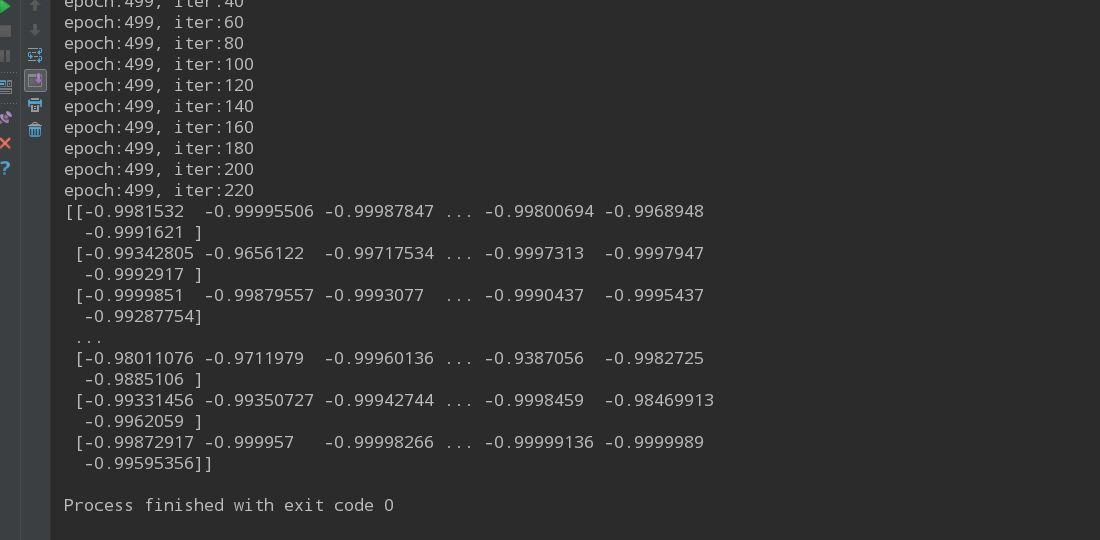训练epoch为300次的实验结果：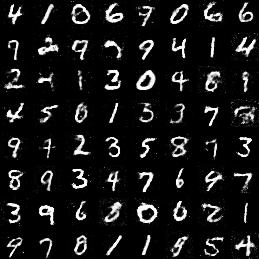生成的和原图像基本一样。

参考：https://blog.csdn.net/z704630835/article/details/82017892
展开全文• 一、原理部分 1.1、 GAN的原理： 1.2、架构 1.3、 GAN 的特点及优缺点： 二、为什么GAN中的优化器不常用SGD 三、为什么GAN不适合处理文本数据 四、训练GAN的一些技巧 ...&am
文章目录一、原理部分1.1、 GAN的原理：1.2、架构1.3、 GAN 的特点及优缺点：二、为什么GAN中的优化器不常用SGD三、为什么GAN不适合处理文本数据四、训练GAN的一些技巧五、GAN的广泛应用

欢迎来到我的目录页：https://blog.csdn.net/Sakura55/article/details/80678611

一、原理部分
首先附上一张流程图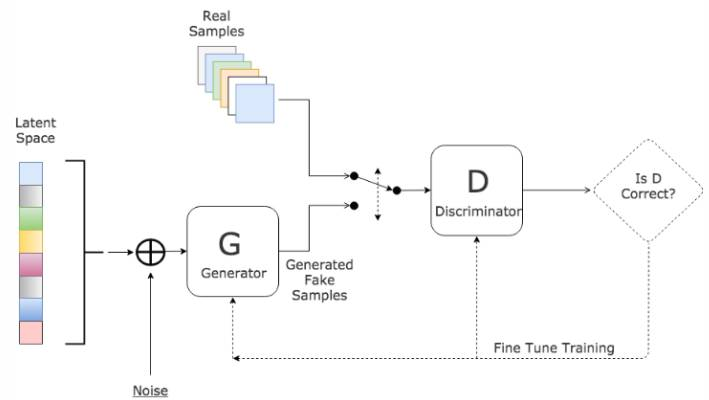1.1、 GAN的原理：
$~~~~~~~~$GAN的主要灵感来源于博弈论中零和博弈的思想，应用到深度学习神经网络上来说，就是**通过生成网络G（Generator）和判别网络D（Discriminator）不断博弈，进而使G学习到数据的分布**，如果用到图片生成上，则训练完成后，G可以从一段随机数中生成逼真的图像。G， D的主要功能是：
$~~~~~~~~$●  G是一个生成式的网络，它接收一个随机的噪声z（随机数），通过这个噪声生成图像
$~~~~~~~~$●  D是一个判别网络，判别一张图片是不是“真实的”。它的输入参数是x，x代表一张图片，输出D（x）代表x为真实图片的概率，如果为1，就代表100%是真实的图片，而输出为0，就代表不可能是真实的图片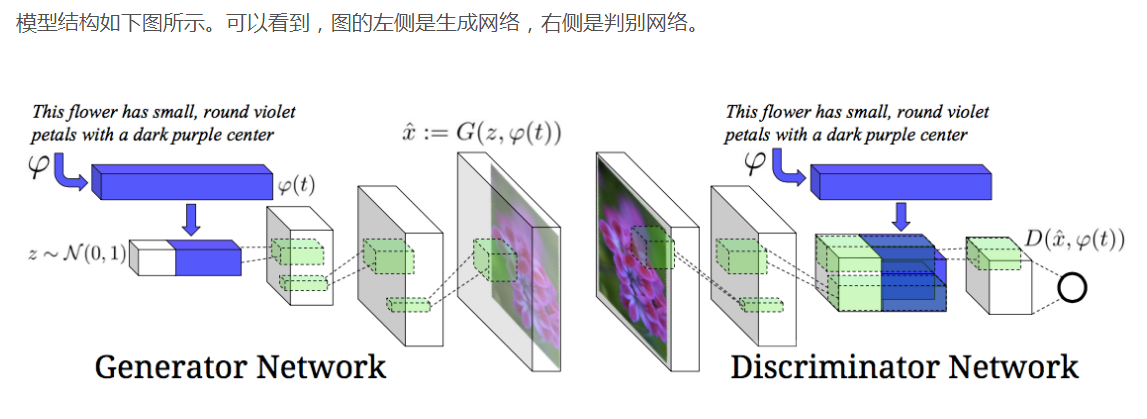$~~~~~~~~$训练过程中，生成网络G的目标就是尽量生成真实的图片去欺骗判别网络D。而D的目标就是尽量辨别出G生成的假图像和真实的图像。这样，G和D构成了一个动态的“博弈过程”，最终的平衡点即纳什均衡点.
1.2、架构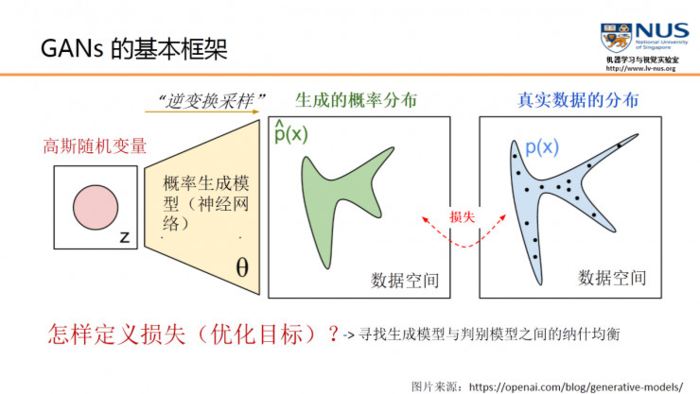$~~~~~~$通过优化目标，使得我们可以调节概率生成模型的参数\theta，从而使得生成的概率分布和真实数据分布尽量接近。
$~~~~~~$那么怎么去定义一个恰当的优化目标或一个损失？传统的生成模型，一般都采用数据的似然性来作为优化的目标，但GAN创新性地使用了另外一种优化目标。首先，它引入了一个判别模型（常用的有支持向量机和多层神经网络）。其次，它的优化过程就是在寻找生成模型和判别模型之间的一个纳什均衡。
$~~~~~~$GAN所建立的一个学习框架，实际上就是生成模型和判别模型之间的一个模仿游戏。**生成模型的目的，就是要尽量去模仿、建模和学习真实数据的分布规律；而判别模型则是要判别自己所得到的一个输入数据，究竟是来自于真实的数据分布还是来自于一个生成模型。**通过这两个内部模型之间不断的竞争，从而提高两个模型的生成能力和判别能力。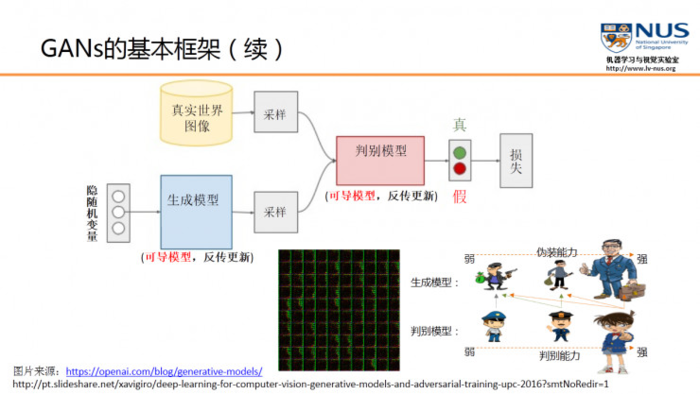$~~~~~~~$当一个判别模型的能力已经非常强的时候，如果生成模型所生成的数据，还是能够使它产生混淆，无法正确判断的话，那我们就认为这个生成模型实际上已经学到了真实数据的分布。
1.3、 GAN 的特点及优缺点：
特点
$~~~~~~~~~~$●  相比较传统的模型，他存在两个不同的网络，而不是单一的网络，并且训练方式采用的是对抗训练方式
$~~~~~~~~~~$●  GAN中G的梯度更新信息来自判别器D，而不是来自数据样本
优点
（以下部分摘自ian goodfellow 在Quora的问答）
$~~~~~~~~~~$●  GAN是一种生成式模型，相比较其他生成模型（玻尔兹曼机和GSNs）只用到了反向传播,而不需要复杂的马尔科夫链
$~~~~~~~~~~$●  相比其他所有模型, GAN可以产生更加清晰，真实的样本
$~~~~~~~~~~$●  GAN采用的是一种无监督的学习方式训练，可以被广泛用在无监督学习和半监督学习领域
$~~~~~~~~~~$●  相比于变分自编码器, GANs没有引入任何决定性偏置( deterministic bias),变分方法引入决定性偏置,因为他们优化对数似然的下界,而不是似然度本身,这看起来导致了VAEs生成的实例比GANs更模糊
$~~~~~~~~~~$●  相比VAE, GANs没有变分下界,如果鉴别器训练良好,那么生成器可以完美的学习到训练样本的分布.换句话说,GANs是渐进一致的,但是VAE是有偏差的
$~~~~~~~~~~$●  GAN应用到一些场景上，比如图片风格迁移，超分辨率，图像补全，去噪，避免了损失函数设计的困难，不管三七二十一，只要有一个的基准，直接上判别器，剩下的就交给对抗训练了。
缺点
$~~~~~~~~~~$●  训练GAN需要达到纳什均衡,有时候可以用梯度下降法做到,有时候做不到.我们还没有找到很好的达到纳什均衡的方法,所以训练GAN相比VAE或者PixelRNN是不稳定的,但我认为在实践中它还是比训练玻尔兹曼机稳定的多
$~~~~~~~~~~$●  GAN不适合处理离散形式的数据，比如文本
$~~~~~~~~~~$● GAN存在训练不稳定、梯度消失、模式崩溃的问题（目前已解决）

附：模式崩溃(model collapse)原因
$~~~~~~~~~~$一般出现在GAN训练不稳定的时候，具体表现为生成出来的结果非常差，但是即使加长训练时间后也无法得到很好的改善。
$~~~~~~~~~~$具体原因可以解释如下：
$~~~~~~~~~~$GAN采用的是对抗训练的方式，G的梯度更新来自D，所以G生成的好不好，得看D怎么说。具体就是G生成一个样本，交给D去评判，D会输出生成的假样本是真样本的概率（0-1），相当于告诉G生成的样本有多大的真实性，G就会根据这个反馈**不断改善自己，提高D输出的概率值**。但是如果某一次G生成的样本可能并不是很真实，但是D给出了正确的评价，或者是G生成的结果中一些特征得到了D的认可，这时候G就会认为我输出的正确的，那么接下来我就这样输出肯定D还会给出比较高的评价，实际上G生成的并不怎么样，但是他们两个就这样自我欺骗下去了，导致最终生成结果缺失一些信息，特征不全。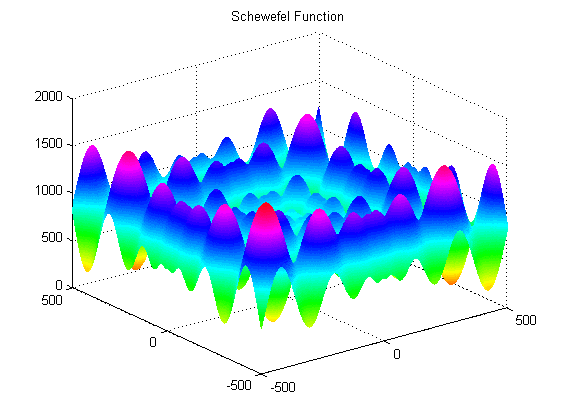$~~~~~~~~~~~~~~~~~~~~~~~~~~~~~~~~~~~~~~~~~$局部极小值点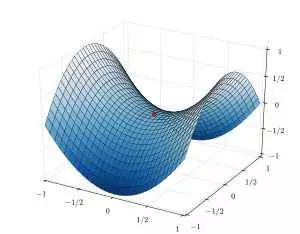$~~~~~~~~~~~~~~~~~~~~~$鞍点
二、为什么GAN中的优化器不常用SGD

SGD容易震荡，容易使GAN训练不稳定，

GAN的目的是在高维非凸的参数空间中找到纳什均衡点，GAN的纳什均衡点是一个鞍点，但是SGD只会找到局部极小值，因为SGD解决的是一个寻找最小值的问题，GAN是一个博弈问题。

三、为什么GAN不适合处理文本数据

文本数据相比较图片数据来说是离散的，因为对于文本来说，通常需要将一个词映射为一个高维的向量，最终预测的输出是一个one-hot向量，假设softmax的输出是（0.2， 0.3， 0.1，0.2，0.15，0.05）那么变为onehot是（0，1，0，0，0，0），如果softmax输出是（0.2， 0.25， 0.2， 0.1，0.15，0.1 ），one-hot仍然是（0， 1， 0， 0， 0， 0），所以对于生成器来说，G输出了不同的结果但是D给出了同样的判别结果，并不能将梯度更新信息很好的传递到G中去，所以D最终输出的判别没有意义。

另外就是GAN的损失函数是JS散度，JS散度不适合衡量不想交分布之间的距离。

（WGAN虽然使用wassertein距离代替了JS散度，但是在生成文本上能力还是有限，GAN在生成文本上的应用有seq-GAN,和强化学习结合的产物）
四、训练GAN的一些技巧

输入规范化到（-1，1）之间，最后一层的激活函数使用tanh（BEGAN除外）

使用wassertein GAN的损失函数，

如果有标签数据的话，尽量使用标签，也有人提出使用反转标签效果很好，另外使用标签平滑，单边标签平滑或者双边标签平滑

使用mini-batch norm， 如果不用batch norm 可以使用instance norm 或者weight norm

避免使用RELU和pooling层，减少稀疏梯度的可能性，可以使用leakrelu激活函数

给D的网络层增加高斯噪声，相当于是一种正则

GAN的变种
自从GAN出世后，得到了广泛研究，先后几百篇不同的GANpaper横空出世，国外有大神整理了一个GAN zoo（GAN动物园），链接如下，感兴趣的可以参考一下：
https://github.com/hindupuravinash/the-gan-zoo
GitHub上已经1200+star了，顺便附上一张GAN的成果图，可见GAN的研究火热程度：
五、GAN的广泛应用

GAN本身是一种生成式模型，所以在数据生成上用的是最普遍的，最常见的是图片生成，常用的有DCGAN WGAN，BEGAN，个人感觉在BEGAN的效果最好而且最简单。

GAN本身也是一种无监督学习的典范，因此它在无监督学习，半监督学习领域都有广泛的应用

不仅在生成领域，GAN在分类领域也占有一席之地，简单来说，就是替换判别器为一个分类器，做多分类任务，而生成器仍然做生成任务，辅助分类器训练。

GAN可以和强化学习结合，目前一个比较好的例子就是seq-GAN

目前比较有意思的应用就是GAN用在**图像风格迁移，图像降噪修复，图像超分辨率了，都有比较好的结果**。

目前也有研究者将GAN用在对抗性攻击上，具体就是训练GAN生成对抗文本，有针对或者无针对的欺骗分类器或者检测系统等等，但是目前没有见到很典范的文章。

参考文献：
https://github.com/hindupuravinash/the-gan-zoo
https://blog.csdn.net/qq_25737169/article/details/78857724#t0
https://www.jianshu.com/p/80bd4d4c2992


展开全文GAN 生成对抗网络 GAN原理
• 假设我们有两个网络，G（Generator）和D（Discriminator）。正如它的名字所暗示的那样，它们的功能分别是： ∙∙\bulletG是一个生成图片的网络，它接收一个随机的噪声z，通过这个噪声生成图片，记做G(z)。 ∙∙\...
一，GAN的原理介绍
$\quad$GAN的基本原理其实非常简单，这里以生成图片为例进行说明。假设我们有两个网络，G（Generator）和D（Discriminator）。正如它的名字所暗示的那样，它们的功能分别是：
$\bullet$G是一个生成图片的网络，它接收一个随机的噪声z，通过这个噪声生成图片，记做G(z)。
$\bullet$D是一个判别网络，判别一张图片是不是“真实的”。它的输入参数是x，x代表一张图片，输出D（x）代表x为真实图片的概率，如果为1，就代表100%是真实的图片，而输出为0，就代表不可能是真实的图片。
$\quad$在训练过程中**，生成网络G的目标就是尽量生成真实的图片去欺骗判别网络D。而D的目标就是尽量把G生成的图片和真实的图片分别开来**。这样，G和D构成了一个动态的“博弈过程”。
$\quad$最后博弈的结果是什么？在最理想的状态下，G可以生成足以“以假乱真”的图片G(z)。对于D来说，它难以判定G生成的图片究竟是不是真实的，因此D(G(z)) = 0.5。
$\quad$这样我们的目的就达成了：我们得到了一个生成式的模型G，它可以用来生成图片。
$\quad$以上只是大致说了一下GAN的核心原理，如何用数学语言描述呢？这里直接摘录论文里的公式：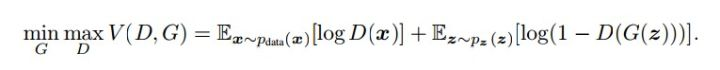简单分析一下这个公式：
$\bullet$整个式子由两项构成。x表示真实图片，z表示输入G网络的噪声，而G(z)表示G网络生成的图片。
$\bullet$D(x)表示D网络判断真实图片是否真实的概率（因为x就是真实的，所以对于D来说，这个值越接近1越好）。而D(G(z))是D网络判断G生成的图片的是否真实的概率
$\bullet$G的目的：上面提到过，D(G(z))是D网络判断G生成的图片是否真实的概率，G应该希望自己生成的图片“越接近真实越好”。也就是说，G希望D(G(z))尽可能得大，这时V(D, G)会变小。因此我们看到式子的最前面的记号是min_G
$\bullet$D的目的：D的能力越强，D(x)应该越大，D(G(x))应该越小。这时V(D,G)会变大。因此式子对于D来说是求最大(max_D)
下面这幅图片很好地描述了这个过程：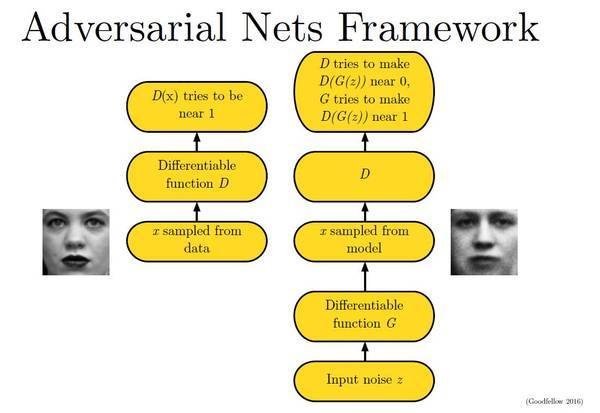那么如何用随机梯度下降法训练D和G？论文中也给出了算法：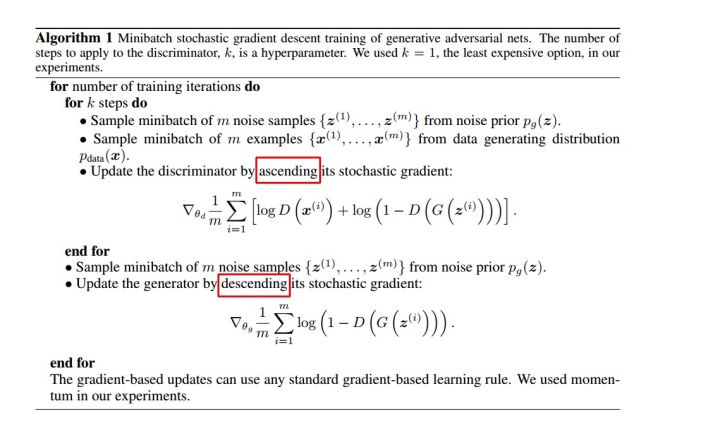这里红框圈出的部分是我们要额外注意的。第一步我们训练D，D是希望V(G,D)越大越好，所以是加上梯度(ascending)。第二步训练G时，V(G, D)越小越好，所以是减去梯度(descending)。整个训练过程交替进行。
$\quad$这个直接看代码就有很好的体现了。
二，DCGAN的原理介绍
$\quad$我们知道深度学习中对图像处理应用最好的模型是CNN，那么如何把CNN与GAN结合？DCGAN是这方面最好的尝试之一，文章最后会附上论文地址：
$\quad$DCGAN的原理和GAN是一样的，这里就不在赘述。它只是把上述的G和D换成了两个卷积神经网络（CNN）。但不是直接换就可以了，DCGAN对卷积神经网络的结构做了一些改变，以提高样本的质量和收敛的速度，这些改变有：
$\bullet$取消所有pooling层。G网络中使用转置卷积（transposed convolutional layer）进行上采样，D网络中用加入stride的卷积代替pooling。
$\bullet$在D和G中均使用batch normalization
$\bullet$去掉FC层，使网络变为全卷积网络
$\bullet$G网络中使用ReLU作为激活函数，最后一层使用tanh
$\bullet$D网络中使用LeakyReLU作为激活函数
$\quad$DCGAN中的G网络示意：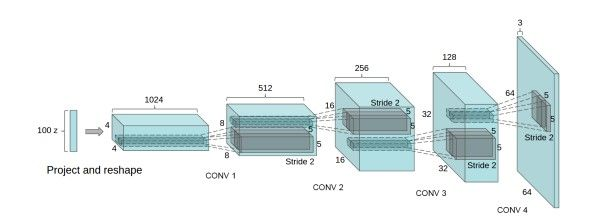$\quad$直接利用1个全连接层实现的GAN如下，补充：一开始的时候生成出来的图像中含有负数以至于无法显示，解决方案是在generate的输出激活函数中不要使用tanh，改为使用sigmoid即可。
$\quad$训练的过程如下：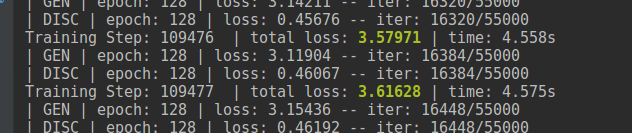训练完成之后生成了20张随机产生的图片，是不是足够以假乱真啦。
#coding=utf-8
import tensorflow as tf
import tflearn
import tflearn.datasets.mnist as mnist
import matplotlib.pyplot as plt
import numpy as np
X, Y, X_test, Y_test = mnist.load_data()
img_dim = 784
z_dim = 200
total_sample = len(X)

#构建生成器和判别器
def generate(x, reuse=tf.AUTO_REUSE):
with tf.variable_scope('Generate', reuse=reuse):
x = tflearn.fully_connected(x,256,activation='relu')
x = tflearn.fully_connected(x,img_dim,activation='sigmoid')
return x
def discriminator(x, reuse=tf.AUTO_REUSE):
with tf.variable_scope('Discriminator', reuse=reuse):
x = tflearn.fully_connected(x, 256, activation='relu')
x = tflearn.fully_connected(x, 1, activation='sigmoid')
return x

#构建网络

gen_input = tflearn.input_data(shape=[None,z_dim], name='input_noise')
disc_input = tflearn.input_data(shape=[None,784], name='disc_input')

#生成器,判别器
gen_sample = generate(gen_input)
disc_real = discriminator(disc_input) #判别网络
disc_fake = discriminator(gen_sample) #欺骗网络D

disc_loss = -tf.reduce_mean(tf.log(disc_real)+tf.log(1. -disc_fake))
gen_loss = -tf.reduce_mean(tf.log(disc_fake))

gen_vars = tflearn.get_layer_variables_by_scope('Generate')
gen_model = tflearn.regression(gen_sample, placeholder=None, optimizer='adam', loss=gen_loss, trainable_vars=gen_vars,batch_size=64,name='target_gen',op_name='GEN')

disc_vars = tflearn.get_layer_variables_by_scope('Discriminator')

gan = tflearn.DNN(gen_model)

#训练并绘制图像
z = np.random.uniform(-1.,1.,[total_sample,z_dim])
gan.fit(X_inputs={gen_input: z,disc_input: X},Y_targets=None,n_epoch=200)

f, a = plt.subplots(2,10,figsize=(10,4))
for i in range(10):
for j in range(2):
#Noise input
z = np.random.uniform(-1.,1.,size=[1,z_dim])
#Generate image from noise. Extend to 3 channels for matplot figure.
temp = [[temp,temp,temp] for temp in list(gan.predict([z]))]
print(temp)
a[j][i].imshow(np.reshape(temp,(28,28,3)))
f.show()
plt.show()


代码2：https://github.com/wiseodd/generative-models
GAN的TF实现
import tensorflow as tf
from tensorflow.examples.tutorials.mnist import input_data
import numpy as np
import matplotlib.pyplot as plt
import matplotlib.gridspec as gridspec
import os

def xavier_init(size):
in_dim = size
xavier_stddev = 1. / tf.sqrt(in_dim / 2.)
return tf.random_normal(shape=size, stddev=xavier_stddev)

X = tf.placeholder(tf.float32, shape=[None, 784])

D_W1 = tf.Variable(xavier_init([784, 128]))
D_b1 = tf.Variable(tf.zeros(shape=))

D_W2 = tf.Variable(xavier_init([128, 1]))
D_b2 = tf.Variable(tf.zeros(shape=))

theta_D = [D_W1, D_W2, D_b1, D_b2]

Z = tf.placeholder(tf.float32, shape=[None, 100])

G_W1 = tf.Variable(xavier_init([100, 128]))
G_b1 = tf.Variable(tf.zeros(shape=))

G_W2 = tf.Variable(xavier_init([128, 784]))
G_b2 = tf.Variable(tf.zeros(shape=))

theta_G = [G_W1, G_W2, G_b1, G_b2]

def sample_Z(m, n):
return np.random.uniform(-1., 1., size=[m, n])

def generator(z):
G_h1 = tf.nn.relu(tf.matmul(z, G_W1) + G_b1)
G_log_prob = tf.matmul(G_h1, G_W2) + G_b2
G_prob = tf.nn.sigmoid(G_log_prob)

return G_prob

def discriminator(x):
D_h1 = tf.nn.relu(tf.matmul(x, D_W1) + D_b1)
D_logit = tf.matmul(D_h1, D_W2) + D_b2
D_prob = tf.nn.sigmoid(D_logit)

return D_prob, D_logit

def plot(samples):
fig = plt.figure(figsize=(4, 4))
gs = gridspec.GridSpec(4, 4)
gs.update(wspace=0.05, hspace=0.05)

for i, sample in enumerate(samples):
ax = plt.subplot(gs[i])
plt.axis('off')
ax.set_xticklabels([])
ax.set_yticklabels([])
ax.set_aspect('equal')
plt.imshow(sample.reshape(28, 28), cmap='Greys_r')

return fig

G_sample = generator(Z)
D_real, D_logit_real = discriminator(X)
D_fake, D_logit_fake = discriminator(G_sample)

# D_loss = -tf.reduce_mean(tf.log(D_real) + tf.log(1. - D_fake))
# G_loss = -tf.reduce_mean(tf.log(D_fake))

# Alternative losses:
# -------------------
D_loss_real = tf.reduce_mean(tf.nn.sigmoid_cross_entropy_with_logits(logits=D_logit_real, labels=tf.ones_like(D_logit_real)))
D_loss_fake = tf.reduce_mean(tf.nn.sigmoid_cross_entropy_with_logits(logits=D_logit_fake, labels=tf.zeros_like(D_logit_fake)))
D_loss = D_loss_real + D_loss_fake
G_loss = tf.reduce_mean(tf.nn.sigmoid_cross_entropy_with_logits(logits=D_logit_fake, labels=tf.ones_like(D_logit_fake)))

mb_size = 128
Z_dim = 100

sess = tf.Session()
sess.run(tf.global_variables_initializer())

if not os.path.exists('out/'):
os.makedirs('out/')

i = 0

for it in range(1000000):
if it % 1000 == 0:
samples = sess.run(G_sample, feed_dict={Z: sample_Z(16, Z_dim)})

fig = plot(samples)
plt.savefig('out/{}.png'.format(str(i).zfill(3)), bbox_inches='tight')
i += 1
plt.close(fig)

X_mb, _ = mnist.train.next_batch(mb_size)

_, D_loss_curr = sess.run([D_solver, D_loss], feed_dict={X: X_mb, Z: sample_Z(mb_size, Z_dim)})
_, G_loss_curr = sess.run([G_solver, G_loss], feed_dict={Z: sample_Z(mb_size, Z_dim)})

if it % 1000 == 0:
print('Iter: {}'.format(it))
print('D loss: {:.4}'. format(D_loss_curr))
print('G_loss: {:.4}'.format(G_loss_curr))
print()

DCGAN待续：
参考链接：
https://zhuanlan.zhihu.com/p/24767059
http://blog.csdn.net/twt520ly/article/details/79420597
https://zhuanlan.zhihu.com/p/27295635
https://wiseodd.github.io/techblog/2016/09/17/gan-tensorflow/
维护了一个微信公众号，分享论文，算法，比赛，生活，欢迎加入。展开全文• 理解自编码的多种变形。 使用对抗神经网络训练模型神经网络 对抗神经网络
• 对抗NN简介 概念介绍 对抗名字的由来及对抗过程 对抗NN的模型 对抗NN的模型和训练 判别网络D的最优值 模拟学习高斯分布 对抗NN实验结果 《生成对抗NN》代码的安装与运行 对抗网络相关论文 论文引用 一、对抗NN...

对抗NN简介
概念介绍
对抗名字的由来及对抗过程
对抗NN的模型
对抗NN的模型和训练
判别网络D的最优值
模拟学习高斯分布
对抗NN实验结果
《生成对抗NN》代码的安装与运行
对抗网络相关论文
论文引用

一、对抗NN简介

大牛Ian J. Goodfellow 的2014年的《Generative Adversative Nets》第一次提出了对抗网络模型，短短两年的时间，这个模型在深度学习生成模型领域已经取得了不错的成果。论文提出了一个新的框架，可以利用对抗过程估计生成模型，相比之前的算法，可以认为是在无监督表示学习(Unsuperivised representation learning)上一个突破，现在主要的应用是用其生成自然图片(natural images)。

二、概念介绍

机器学习两个模型——生成模型和判别模型。

生成模型(Generative):学习到的是对于所观察数据的联合分布 比如2-D: p(x,y).
判别模型:学习到的是条件概率分布p(y|x),即学习到的是观察变量x的前提下的非观察变量的分布情况。

通俗的说，我们想通过生成模型来从数据中学习到分布情况，来生成新的数据。比如从大量的图片中学习，然后生成一张新的Photo.
而对于判别模型，最经典的应用，比如监督学习，那么对于分类问题，我想知道输入x，输出y的情况，那么y的值可以理解为数据的label。

而其中的对抗神经网络就是一个判别模型(Discriminative, D)和一个生成模型(Generative ,G)的组成的。

三、对抗名字的由来及对抗过程

刚才介绍了对抗网络其实是一个D和一个G组成的，那么G和D之间是如何对抗的呢？
先看以下一个场景:

D是银行的Teller
G是一个Crook，专门制造假币。

那么其中的对抗过程就是，对于D来说，不断的学习，来进行真币的判断，G则是不断学习，制造更像真币的假币，来欺骗D，而最后的训练结果则是——D可以很好的区分真假币，但是G制造了“如假包换”的假币，而D分辨不出。

而对于对抗网络来说，D和G都是一个神经网络模型——MLP,那么D(判别模型)的输出是一个常量，这个常量表示“来自真币”的可能性。而对于G的输出则是一组向量，而这个向量表示的就是”假币”。

四、对抗NN的模型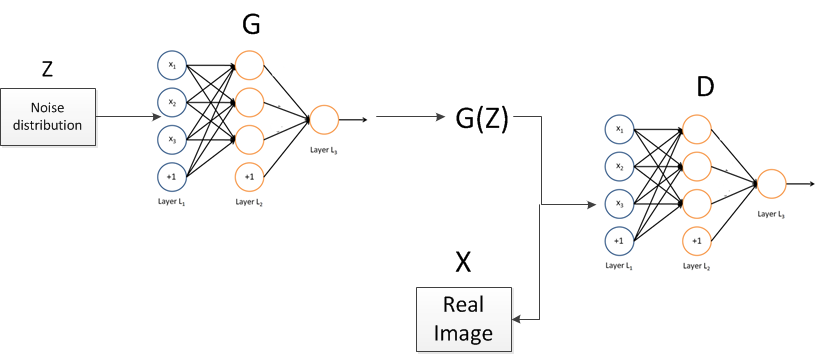图片1

图片1中的Z是G的输入，一般情况下是高斯随机分布生成的数据；其中G的输出是G(z),对于真实的数据，一般都为图片，将分布变量用X来表示。那么对于D的输出则是判断来自X的可能性，是一个常量。

五、对抗NN的训练和优化

对于G来说，要不断的欺骗D，那么也就是:

max log(D(G(z)))                           目标函数1


对于D来说，要不断的学习防止被D欺骗，那么也就是:

max log(D(x)) + log(1 - D(G(z)))           目标函数2


使用梯度下降法(GD)训练，那么梯度如下。

对于目标函数1来说: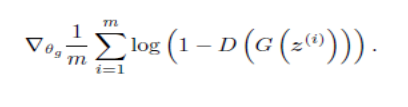对于目标函数2来说: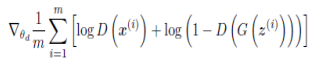训练过程

论文给出了Algorithm 1,详细内容请查看原文，就是先进行训练D，然后训练G。其中论文也给出了公式来证明算法的可收敛性。

训练的几个trick:

论文提到的dropout的使用(应该是maxout layer)
每次进行多次D的训练，在进行G的训练，防止过拟合。
在训练之前，可以先进行预训练。

六、判别网络D的最优值

将X的概率密度分布函数(pdf)定义为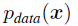将G(Z)的pdf定义为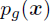那么对于每一次训练，G如果固定的话，最优的输出D的值可以认为是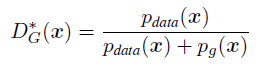而且，最后训练的结果，是D=1/2=0.5。即此时有: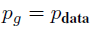关于此详细证明可以查看原文。

七、对抗NN的实验结果

论文1用到的数据集包括，MNIST a)、TFD b)、CIFAR-10 c) d)，数据集。对于不同的数据集，原文用到了不同的网络模型。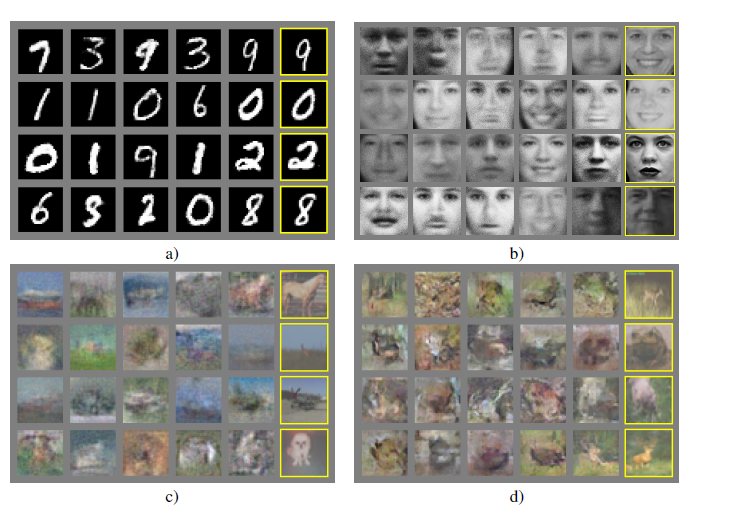图片2-实验结果

模型如下。

数据集
G模型
D模型

mnist
relu+sigmoid 激活函数
maxout+sigmoid

tfd
没有提到
没有提到

CIFAR-10 c）
全连接+激活函数
maxout+sigmoid

CIFAR-10 d）
反卷积层+激活函数
maxoutconv+sigmoid

详细模型介绍请查看开源项目中的yaml文件

八、模拟学习高斯分布

论文给出的一张图。如下: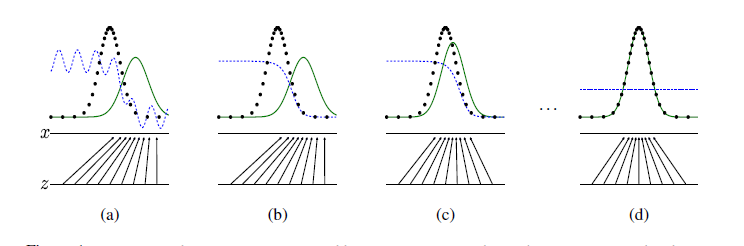D , blue , dashed line
X , black , dotted line
G , green , solid line

其中是通过对抗网络，让G(z)学习到x的分布，而x是符合高斯分布的，z是均匀分布。其中从(a)到(d)就是不断学习的过程，刚开始，G(z)和X的pdf是不吻合的，因为刚开始G(z)不可能一下就从随机变量中生成目标分布的数据。不过，最后，我们也可以看到(d)是最后学习到图像，其中下边两条平行线，z经过G()的映射已经和x的分布完全吻合(当然这是一个理想的情况)，而且，D的输出是一条直线，就像上文提到的，D() = 1/2 一个常量。

Tensorflow 相关代码

(1)Discriminator’s loss

batch=tf.Variable(0)
obj_d=tf.reduce_mean(tf.log(D1)+tf.log(1-D2))
.minimize(1-obj_d,global_step=batch,var_list=theta_d)


(2)Generator’s loss

batch=tf.Variable(0)
obj_g=tf.reduce_mean(tf.log(D2))
.minimize(1-obj_g,global_step=batch,var_list=theta_g)

(3)Training Algorithms 1 , GoodFellow et al. 2014

for i in range(TRAIN_ITERS):
x= np.random.normal(mu,sigma,M)
z= np.random.random(M)
sess.run(opt_d, {x_node: x, z_node: z})    //先训练D
z= np.random.random(M)
sess.run(opt_g, {z_node: z})               //在训练G

以上代码是Tensorflow实现的用对抗NN生成高斯分布的例子。

九、大牛Good fellow 论文代码的安装与运行

对抗网络的作者Goodfellow也开源了自己的代码。

(1)项目链接

(2)下载与依赖库的安装

项目依赖pylearn2 ，要先安装pylearn2

export PYLEARN2_VIEWER_COMMAND="eog --new-instance"
export PYLEARN2_DATA_PATH=/home/data
export PYTHONPATH=/home/code


其他python 依赖库可以通过pip或者apt-get安装。

(3)训练和测试

调用pylearn2的 train.py 和mnist.yaml进行训练。

pylearn2/scripts/train.py ./adversarial/mnist.yaml

测试如下

python show_samples_mnist_paper.py mnist.pkl

十、对抗网络相关论文和应用

博主做了一个开源项目，收集了对抗网络相关的paper和论文。
欢迎star和Contribution。

对抗NN的应用。这些应用都可以从我的开源项目中找到。

(1)论文其中使用了CNN，用于图像生成，其中将D用于分类，取得了不错的效果。

(2)论文将对抗NN用在了视频帧的预测，解决了其他算法容易产生fuzzy 块等问题。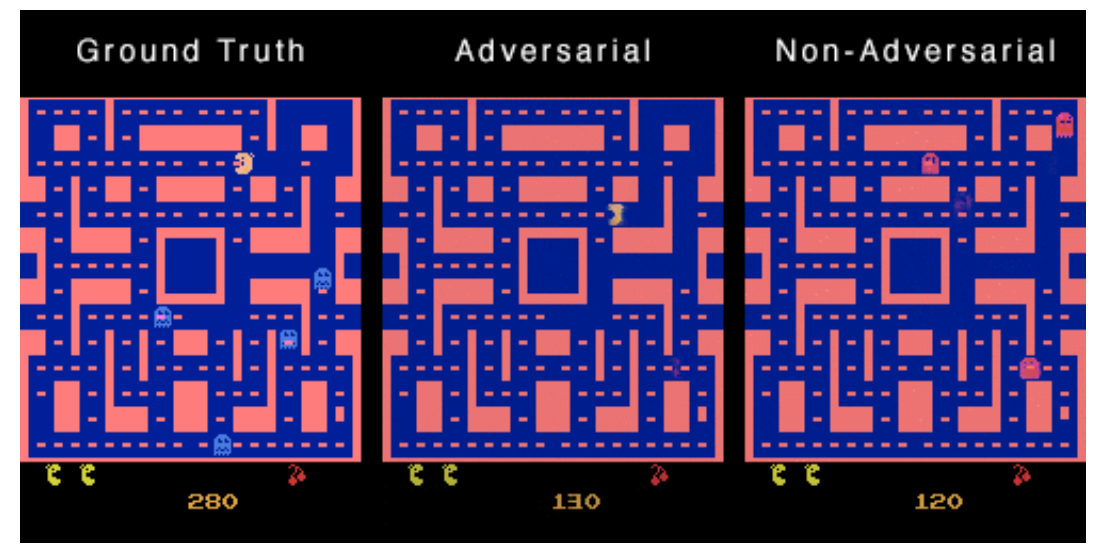(3)论文将对抗NN用在了图片风格化处理可视化操作应用上。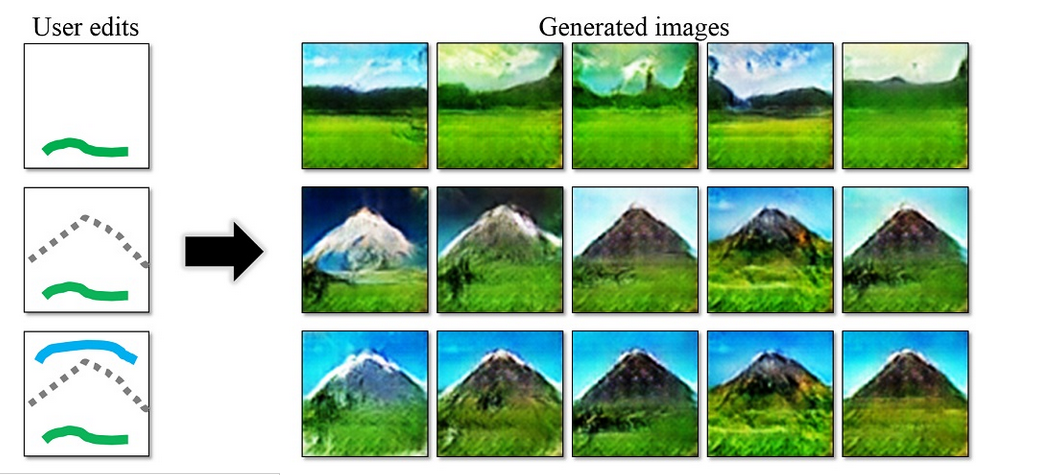十一、论文引用

Deep multi-scale video prediction beyond mean square error.Michael Mathieu.
Generative Visual Manipulation on the Natural Image Manifold.Jun-Yan Zhu.ECCV 2016.`
展开全文深度学习 对抗神经网络 神经网络 caffe
• ## 对抗神经网络

千次阅读 2018-08-20 11:40:28
对抗NN简介 概念介绍 对抗名字的由来及对抗过程 对抗NN的模型 对抗NN的模型和训练 判别网络D的最优值 模拟学习高斯分布 ... 对抗网络相关论文 论文引用 一、对抗NN简介 大牛Ian J. Goodfellow 的20...
• I organized this reposity mainly for learning GANs, so all codes about classical GANs were implemented with simple network structure and tested by MNIST dataset. Just know about how mathmatical ana
• 这是一个稍微更新的模型，用于“学习用对抗神经网络加密来保护通信”的论文。我们想神经网络是否可以学习使用秘密密钥来保护来自其他神经网络的信息。具体来说，我们专注于确保多代理系统中的机密属性，并且我们根据...神经网络 人工智能 加密
• 生成对抗神经网络（GAN）中有两个模型，一个是生成器（Generator），另一个是判别器（Discriminator）。生成器希望生成一个近似真实的图片（也可以不是图片，根据不同的神经网络结构，生成对抗神经网络可以被用来...
• 本资源是机器学习 台大教授 李宏毅对抗神经网络GAN是一门多领域交叉学科，涉及概率论、统计学、逼近论、凸分析、算法复杂度理论等多门学科。宏毅的授课配PPT讲稿。
• 深度卷积对抗神经网络（DCGAN) 从随机向量生成真实图像 如何构建一个新的GAN网络 1.定义目标 2.定义G的输入输出 3.定义D的输入输出 4.设计G和D的结构 目标函数 最大化D(是否是真的概率）,最小化G 训练判别器，...
• 对抗神经网络其实是两个网络的组合，可以理解为一个网络生成模拟数据，另一个网络判断生成的数据是真实的还是模拟的。生成模拟数据的网络要不断优化自己让判别的网络判断不出来，判别的网络也要优化自己让自己判断得...
• 对抗神经网络模型(GANs)作为当下最火的神经网络模型神经网络 机器学习
• 在文章中，作者提出了一种新颖的基于生成对抗神经网络的协同过滤推荐技术，可以获得更高的推荐系统准确性。具体地；文章通过一系列方法解决了传统IRGAN存在的问题，同时针对协同过滤也进行了优化。 协同过滤推荐 ...人工智能 推荐系统 深度学习
• 上一篇博文简单介绍了对抗网络的理论和大概流程。其中所谓的对抗网络可以归结为unsupervised learning 或者 generative model。从无监督学习来进行feature representation,有k-means聚类算法，auto-encoders，...神经网络 对抗神经网络 深度学习 tensorflow
• 本人作为IT从业者，学习对抗神经网络，以IT视角描述GANs，并使用开源代码资源学习GANs，与实际数据不足工作需求结合，基于Keras框架，通过调整DCGAN网络结构和参数，生成可以以假乱真的数据样本图片。并记录了中间...DCGAN 深度学习 Python Keras